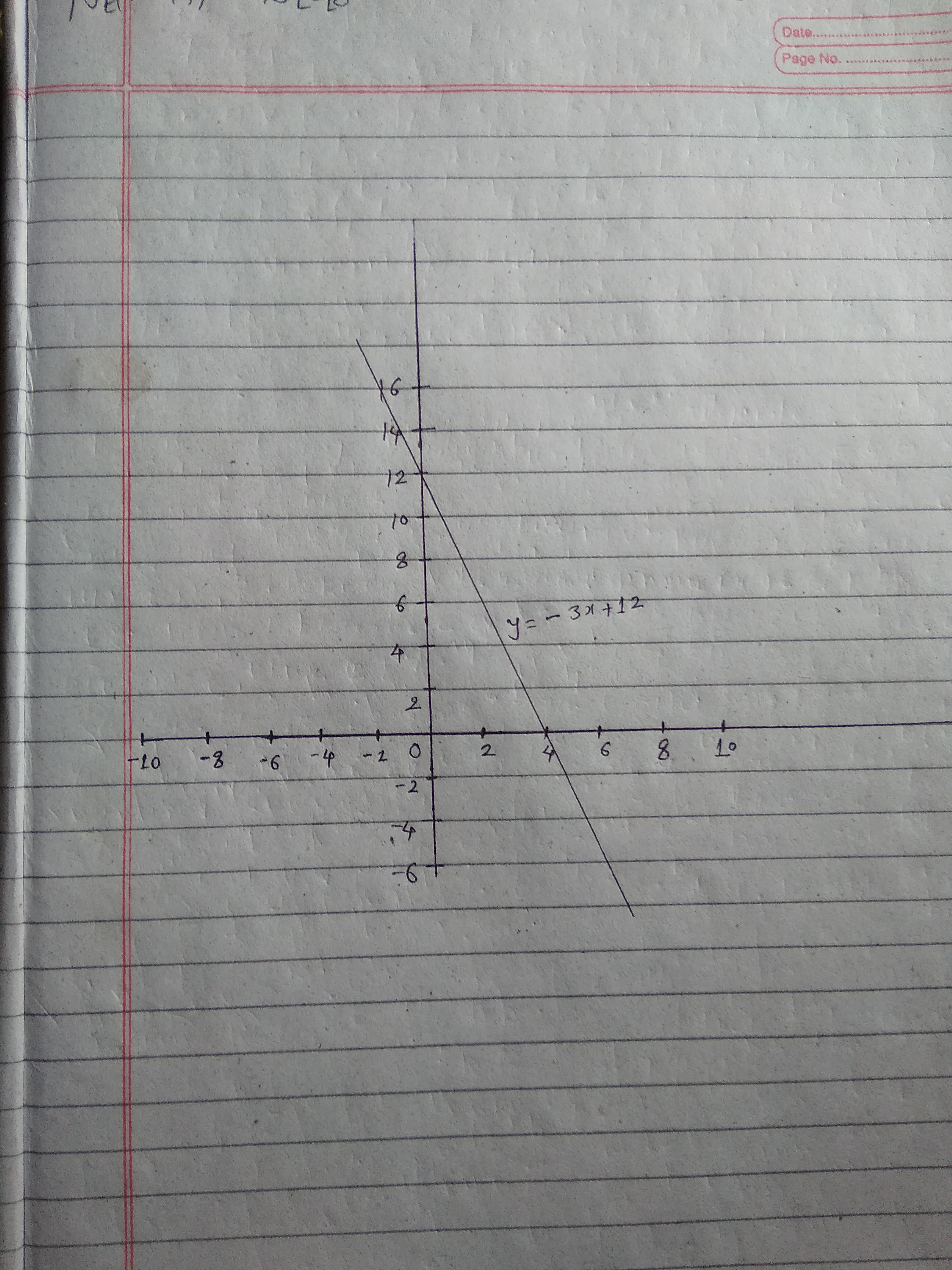Question: Does the zero vector have no basis? Justify . Construct the real polynomial degree at most 2 whose graph contaions the point $$(-4 , 24) ,(1 , 9)\ and\ (3 , 3)$$ sketch the graph.

Solution:
First Part:
No,empty set the basis for zero vector.
Second part:
Here $$c_0 = -4, c_1 = 1, c_2 = 3$$ and $$b_0 = 24 , b_1 = 9 , b_2 = 3$$
Here degree is at most 2 ,so n = 2
Now we find
$$F_0(x) = \prod_{(k =0, k \neq 0)}^{2}$$
$$F_0(x)=\frac{x - ck}{c0 - ck}$$\ =$$\frac{(x - c_1)(x - c_2)}{(c_0 - c_1)(c_0 - c_1)}$$
= $$\frac{(x-1)(x-3)}{(-4-1)(-4-3)}$$
=$$\frac{x^2 - 4x + 3}{35}$$
$$F_1(x)$$ = $$∏_{(k=0, k ≠1)}^{2}\frac{(x-ck)}{(c1-ck)}$$
= $$\frac{(x - c_o)(x - c_2)}{(c_1 - c0)(c_1 - c_2)}$$
= $$\frac{(x + 4)(x - 3)}{(1 + 4)(1 - 3)}$$
=$$\frac{(x_2 + x - 12)}{-10}$$
$$F_2(x) = \prod_{(k=0, k ≠2)}^{2}\frac{x - ck}{c_2 - c_k}$$
=$$\frac{(x + 4)(x - 1)}{(3 + 4)(3 - 1)}$$
=$$\frac{(x^2 + 3x - 4)}{14}$$
Hence the require polynomial is
$$F(x) = \sum_{(i = 0)}^{2}b_if_i(x) = b_0F_0(x) + b_1f_1(x) + b_2f_2(x)$$
$$F(x) = 24 \frac { (x_2 - 4x + 3)}{35} + 9 \frac{(x^2 + x - 12)}{(-10)} +3 \frac{(x^2 + 3x - 4)}{14}$$

$F(x) = \frac{48(x^2 - 4x + 3)- 63(x^3 + x -12) + 15(x^2 + 3x - 4)}{70} \\ or,\ F(x) = \frac{48x^2 - 192x + 144 - 63x^2 -63x + 756 + 15x^2 + 45x - 60}{70} \\ or,\ F(x) = \frac{45x - 255x + 900 - 60}{70} \\ or,\ F(x) = \frac{(-210 + 840)}{70} \\ or,\ F(x) = -3x + 12 \\$Tags:

Categories:

Updated: